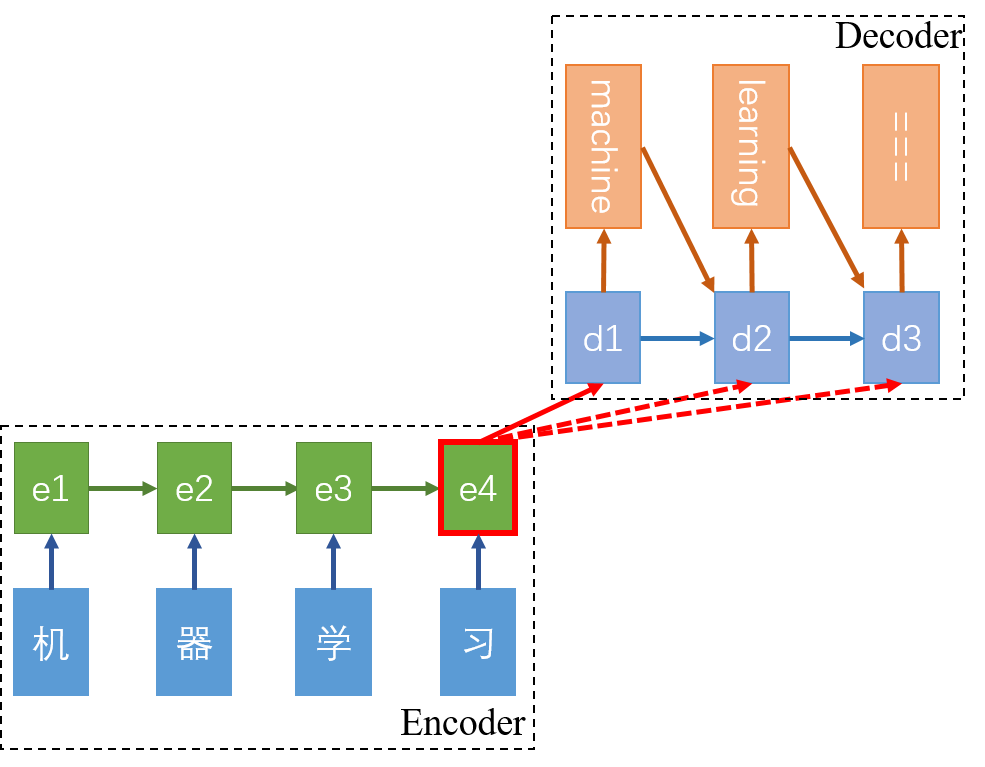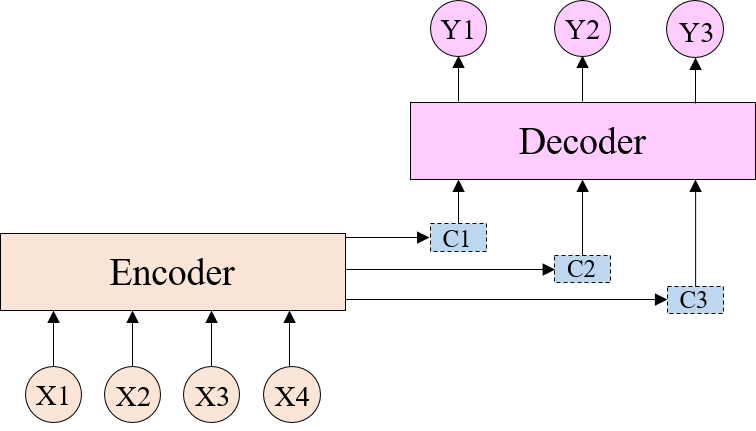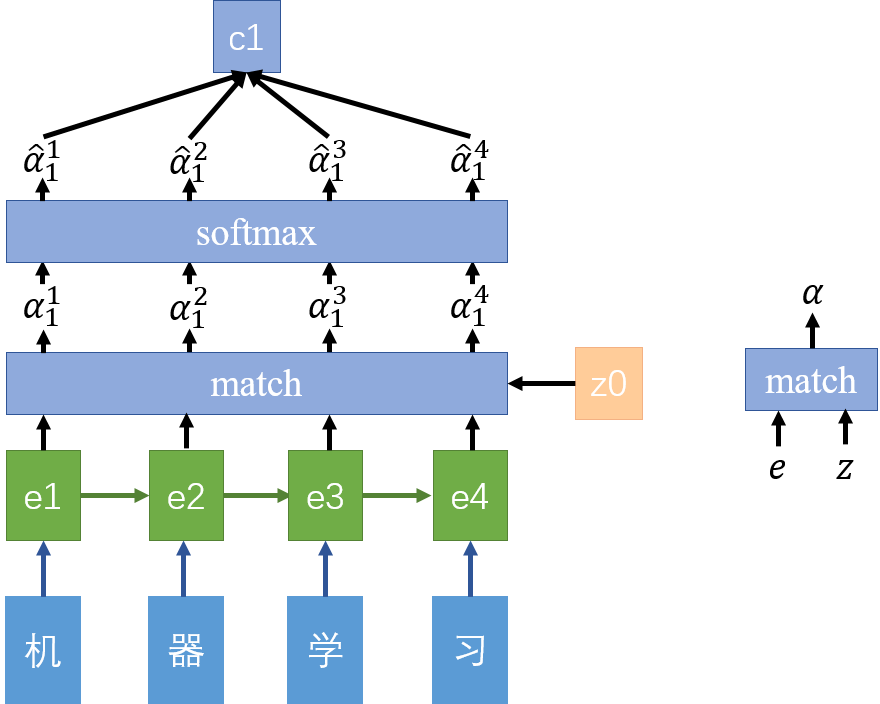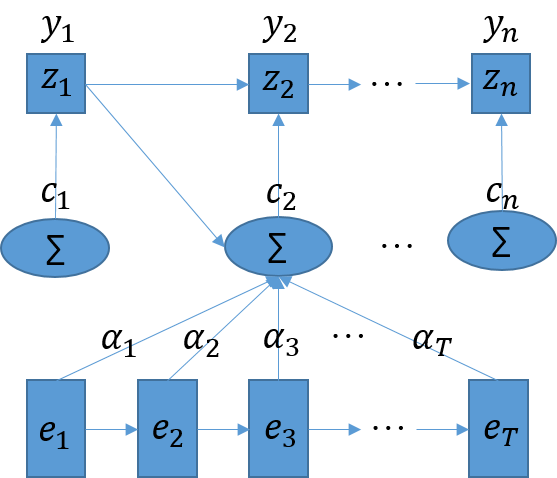Attention机制A potential issue with this encoder–decoder approach is that a neural network needs to be able to compress all the necessary information of a source sentence into a fixed-length vector. This may make it difficult for the neural network to cope with long sentences, especially those that are longer than the sentences in the training corpus.

Attention机制1. 首先，$e_1$$z_0$一起输入match模块，得到$\alpha_1^1$，同理，$e_2$$e_3$$e_4$分别和$z_0$输入match模块也能得到$\alpha_1^2$$\alpha_1^3$$\alpha_1^4$。match模块可以看作是在计算$e$$z$的相似度，如上图右边所示。$z$是Decoder模块的隐含层变量，因此这里$z_0$是隐含层的初始值。match模块常有三种选择：1）直接计算向量的余弦相似度；2）一个小的神经网络模型，输入是$e$$h$，输出是一个标量；3）$\alpha=e^TWz$。采用（2）和（3）两种方式时，match模块的参数也是随着网络反向传播一同学习的。
2. 计算出Encoder每一个隐变量$e$$z$的相似度后，需要将其归一化，常采用softmax函数，归一化后得到的$\hat\alpha$可以看作是Decoder在输出$Y1$时对Encoder中每一个隐含层信息的关注度。
3. 最后对隐含层信息求加权和即可得到$c_1$
$c_1=\sum\hat\alpha_1^ie_i$

Attention机制的计算公式可以整理如下：
$\alpha_t^i=match(z_{t-1},e_i)=V\cdot tanh(W\cdot e_i+U\cdot z_{t-1}+b)\\ \hat\alpha_t^i=\frac{exp(\alpha_t^i)}{\sum_{k=1}^{T_x}exp(\alpha_t^k)}\\ c_t=\sum_{k=1}^{T_x}\hat\alpha_1^ke_k\\ z_t=f(z_{t-1},y_{t-1},c_t)$总结

Attention机制几乎是现在所有序列学习的必备，Attention不是一种模型而是一种思想，受到人类在处理数据时分散注意力的启发，许多模型加上Attention机制都得到了state-of-art的结果，也促成了该思想的蓬勃发展。